# 1.介绍

RNN是神经序列模型，并在多个重要任务上达到了state-of-the-art的效果。我们知道，神经网络的成功应用需要好的正则化（regularization）。不幸的是，对于前馈神经网络最重要的regularzation：dropout(Srivastava 2013)，却不能与RNNs有效运行。因而，RNNs的实际应用经常使用很小的模型，因为大的RNNs会趋向于overfit。已经存在的regularization方法对于RNNs的相对提升较小。在本文中，我们展示了正确使用dropout时可以极大减小在LSTMs中的overfitting，并在三个不同问题上进行了evaluate。

# 3.LSTM cells RNN的正则化

• $h_t^l \in R^n$是在timestep t时在layer l上的一个hidden state。
• $T_{n,m}: R^n \rightarrow R^m$是一个仿射变换（Wx+b, 对于一些W和b）。
• $\odot$是element-wise乘法，
• $h_t^0$是一个在timestep t上的input word vector。

## 3.1 LSTM units

RNN的动态性可以使用从前一hidden states到当前hidden states上的确定转换（deterministic transitions）来描述。deterministic state transition是一个函数：

$RNN: h_t^{l-1}, h_{t-1}^l \rightarrow h_t^l$

$h_t^l = f(T_{n,n} h_t^{l-1} + T_{n,n} h_{t-1}^l), where \ f \in \lbrace sigm, tanh \rbrace$

LSTM具有复杂的动态性，使得它对于可以轻易地“记住（memorize）” 一段时间内的信息。“long term” memory被存储在memory cells $c_t^l \in R^n$的一个vector中。尽管许多LSTM的架构在它们的连接结构和激活函数上有所区别，所有的LSTM结构都具有显式的memory cells来存储长期的信息。LSTM可以决定是否overwrite memory cell，或者检索它，或者在下一time step上继续保留它。LSTM的结构如下：

$LSTM: h_t^{l-1}, h_{t-1}^l, c_{t-1}^l \rightarrow h_t^l,c_t^l \\ \begin{pmatrix} i \\ j \\ o \\ g \end{pmatrix} = \begin{pmatrix} sigm \\ sigm \\ sigm \\ tanh \end{pmatrix} T_{2n, 4n} \begin{pmatrix} h_t^{l-1} \\ h_{t-1}^l \end{pmatrix} \\ c_t^l = f \odot c_{t-1}^l + i \odot g \\ h_t^l = o \odot tanh(c_t^l)$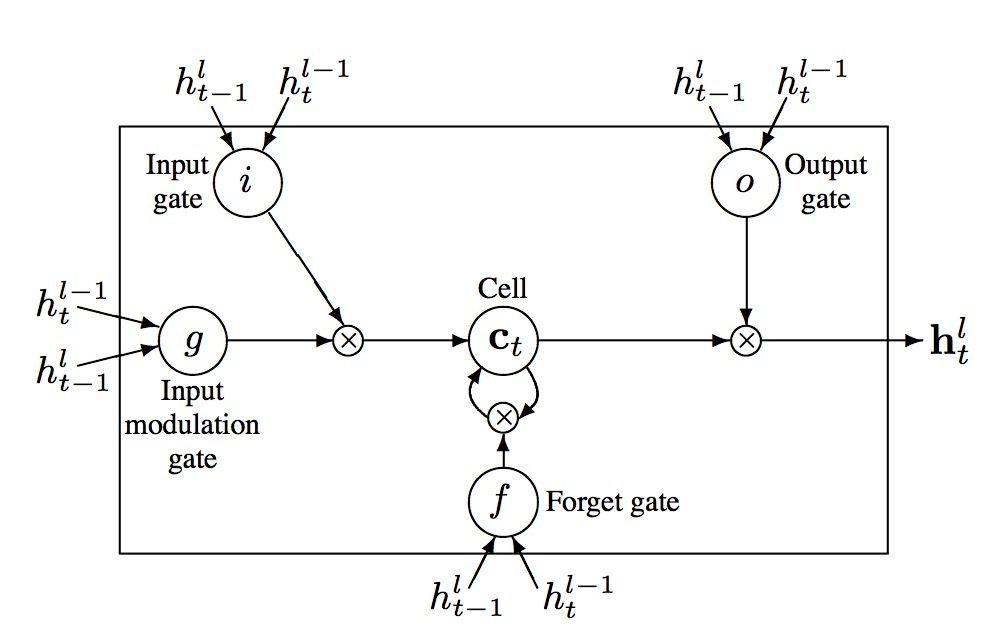## 3.2 使用dropout正则化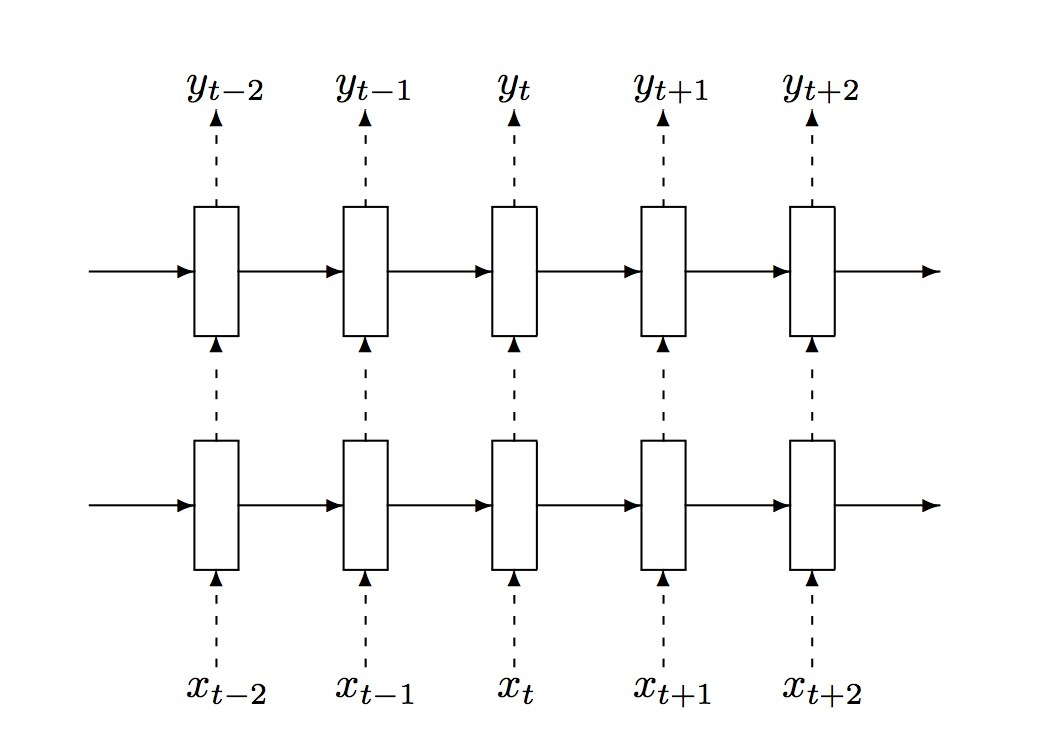$\begin{pmatrix} i \\ j \\ o \\ g \end{pmatrix} = \begin{pmatrix} sigm \\ sigm \\ sigm \\ tanh \end{pmatrix} T_{2n, 4n} \begin{pmatrix} D(h_t^{l-1}) \\ h_{t-1}^l \end{pmatrix} \\ c_t^l = f \odot c_{t-1}^l + i \odot g \\ h_t^l = o \odot tanh(c_t^l)$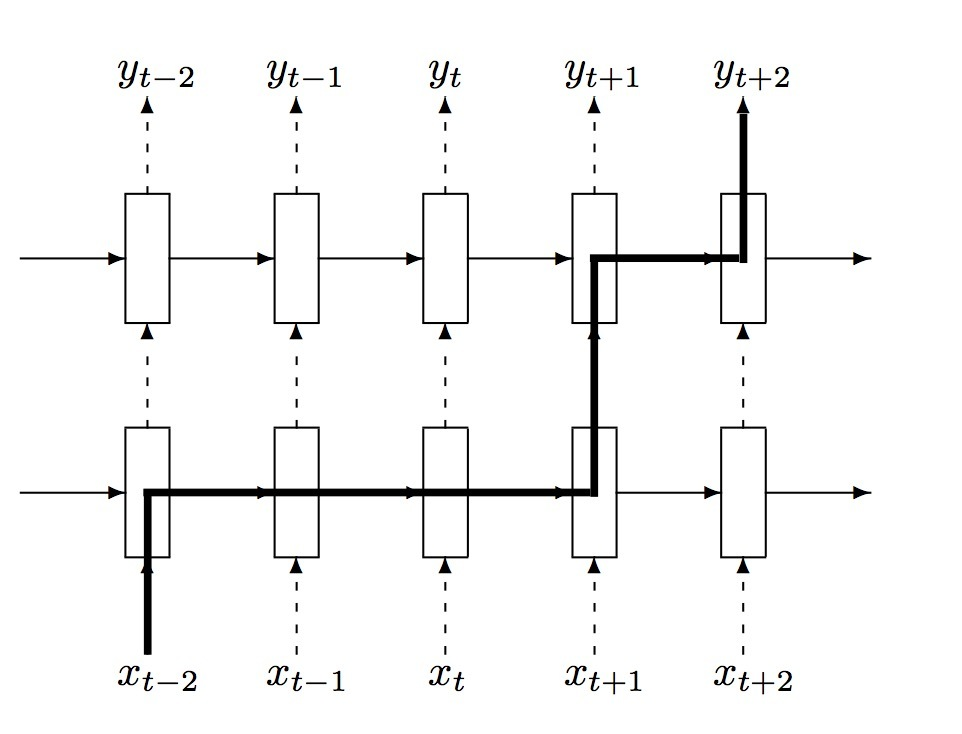# xavier initialization介绍

Xavier Glorot在2010年的《Understanding the difficulty of training deep feedforward neural networks》提出了xavier glorot intialization，该方法在tensorflow中直接有集成。

# 2.实验设置和数据集

## 2.2 有限数据集

• MNIST digits数据集：50000训练图片、10000验证图片、10000测试图片、每个展示了一个关于10个数字的28x28灰度图片。

• CIFAR-10: 50000训练样本、10000验证图片、10000测试图片。10个类，每个对应于图片上的一个物体：飞机、汽车、鸟、等等。

• Small-ImageNet：

## 2.3 实验设置

$W_{ij} ~ U[\frac{1}{\sqrt{n}}, \frac{1}{\sqrt{n}}]$

# 4.研究梯度和它们的传播

## 4.2 初始化时的梯度

### 4.2.1 一种新的归一化初始化理论思考



…(2)

…(3)

…(4)

…(5)

…(6) …(7)

…(8)

…(9)

…(10)

…(11)

…(12)

…(13)

…(14)

$n Var[W] = \frac{1}{3}$

…(15)

$W \sim U [ - \frac{\sqrt{6}}{\sqrt{n_j + n_{j+1}}}, \frac{\sqrt{6}}{\sqrt{n_j + n_{j+1}}} ]$

…(16)

### 4.2.2 梯度传播研究

$J^i = \frac{\partial z^{i+1}}{\partial z^i}$

…(17)

# Faster R-CNN介绍

Faster R-CNN由Ross Girshick等人提出。

# 总览RPN被设计成使用一个较广范围的比例（scales）和高宽比（aspect ratios）来高效地预测region proposals。对比于上面使用图片金字塔（图1，a）的方法或者过滤器金字塔（图1，b），我们引入了新的锚点边框“anchor” boxes，在多个不同尺度和高宽比的情况下充当参照（references）。我们的scheme可以被看成是一个对参照（references）进行回归的金字塔（图1,c），它可以避免枚举多个不同尺度和高宽比的图片或filters。当使用单尺度图片进行训练和测试时，该模型执行很好，并且能提升运行速度。

# 2.相关工作

• 基于grouping super-pixels的方法（Selective Search, CPMC, MCG）
• 基于滑动窗口的方法（objectness in windows, EdgeBoxes )。

Object proposal methods被看成是dectectors之外独立的一个模块。

。。。

# 3.Faster R-CNN

## 3.1 RPN

### 3.1.1 Anchors

#### 平移不变的Anchors### 3.1.2 Loss函数

• (i) 具有与一个ground truth box的IoU（Intersection-over-Union）重合率最高的anchor/anchors
• (ii) 具有一个与任意ground-truth box的IoU重合度高于0.7的anchor

$L(\{p_i\}, \{t_i\}) = \frac{1}{N_{cls}} \sum_{i} L_{cls} (p_i, p^*) + \lambda \frac{1}{N_{reg}} \sum_i p_i^* L_{reg}(t_i, t_i^*)$ …(1)

• i表示在mini-batch中的一个anchor的索引
• $p_i$表示anchor i是object的预测概率。
• 如果该anchor为正，ground-truth label $p_i^*$是1;否则为0.
• $t_i$是一个向量，表示要预测的bounding box的4个参数化坐标
• $t_i^*$是与一个正锚点（positive anchor）相关的ground-truth box。
• $L_{cls}$是分类loss，它是关于两个类别的log loss。
• $L_{reg}(t_i, t_i^*) = R (t_i - t_i^*)$是回归loss，其中R是robust loss function（L1平滑）。
• $p_i^* L_{reg}$意味着regression loss当为正锚点时（$p_i^*=1$）激活，否则禁止（$p_i^*=0$）

$t_x = (x-x_a) / w_a, t_y = (y-y_s) / h_a$ $t_w = log(w/w_a), t_h = log(h/h_a)$ $t_x^*=(x^* - x_a)/w_a, t_y^*=(y^* - y_a) / h_a$ $t_w^* = log(w^*/w_a), t_h^* = log(h^*/h_a)$

…(2)

### 3.1.3 训练RPNs

RPN可以通过BP和SGD以end-to-end的方式进行训练。我们根据”以图片为中心（image-centric）”的抽样策略来训练网络。从单个图片中提取的每个mini-batch，包含了许多正负样本锚点。它可以为所有anchors的loss functions进行优化，但会偏向主导地位的负样本。作为替代，我们在一个图片上随机抽取256个锚点，来计算一个mini-batch的loss函数，其中抽样到的正负锚点的比例为1:1。如果在一个图片中正样本数少于128个，我们将将该mini-batch以负样本进行补齐。

## 3.2 为RPN和Rast R-CNN共享特征

• (i) 交替训练（Alternating training）。在这种方案中，我们首先训练RPN，接着使用这些proposals来训练Fast R-CNN。 该网络会通过Fast R-CNN进行调参，接着被用于初始化RPN，然后反复迭代该过程。这种方案被用于该paper中的所有实验。
• (ii) 近似联合训练（Approximate joint training）。在这种方案中，RPN和Fast R-CNN网络在训练期间被合并到一个网络中，如图2所示。在每个SGD迭代过程中，forward pass会生成region proposals（当训练一个Fast R-CNN detector时，他们被看成是固定的、预计算好的proposals）。backward propagation会和往常一样进行，其中对于共享的layers来说，来自RPN loss的Fast R-CNN loss的后向传播信号是组合在一起的。该方案很容易实现。但该方案会忽略到关于proposal boxes坐标的导数（derivative w.r.t. the proposal boxes’ coordinates）, 也就是网络响应，因而是近似的。在我们的实验中，我们期望发现该求解会产生闭式结果，并减少大约25-50%的训练时间（对比alternating training）。该求解在python代码中包含。
• (iii) 非近似联合训练（Non-approximate joint training）。根据上述讨论，由RPN预测的bounding boxes也是输入函数。在Fast R-CNN中的RoI pooling layer会接受conv features，以及预测的bounding boxes作为输入，因而一个理论合理的BP解也与box坐标的梯度有关。这些梯度在上面的approximate joint training会被忽略。在非近似方法中，我们需要一个RoI pooling layer，它是box坐标的微分。这是一个非平凡问题，解可以通过一个”RoI warping” layer给出。（超出本paper讨论范围）

# 4.实验

## 4.1 PASCAL VOC

RPN上的Ablation实验。为了研究RPN作为proposal method的行为，我们做了一些ablation研究。首先，我们展示了在RPN和Fast R-CNN检测网络间共享卷积层（conv layers）的效果。为了达到这个，我们在第二个step后停止训练过程。使用独立的网络将结果减小到58.7%（RPN+ZF,unshared, 表2）。我们观察到这是因为在第三个step中，当detector-tuned features被用于fine-tune该RPN时，proposal质量会被提升。

# BlazingText介绍

Amazon在hogwild、hogbatch之后，提出了《BlazingText: Scaling and Accelerating Word2Vec using Multiple GPUs 》，利用多GPU来加速word2vec的训练。

# 介绍

word2vec的最优化通过SGD完成，它会进行迭代式求解；在每一个step，会选取一个词对（pair of words）：一个输入词和一个目标词，它们来自于window或一个随机负样本。接着根据选中的两个词来计算目标函数的梯度，然后基于该梯度值更新两个词的词表示（word representations）。该算法接着使用不同的word pair来处理下一次迭代。

SGD的一个主要问题是，它的顺序性；这是因为它在这一轮迭代的更新、与下一轮迭代的计算之间存在着依赖关系（他们可能遇到相同的词表示），每一轮迭代必须潜在等待前一轮迭代的更新完成。这不允许我们使用硬件并行资源。

Hogwild方法在多核架构上的成功，使得该算法利用GPU成为可能，GPU比CPU提供更强的并行化。在该paper中，我们提出了一种有效的并行化技术来使用GPU加速word2vec。

# 2.word2vec模型

word2vec有两种不同的模型架构：Contextual Bag-Of-Words (CBOW)以及Skip-Gram with Negative Sampling (SGNS) 。CBOW的目标函数是由给定上下文去预测一个词，而Skipgram则给定一个词去预测它的上下文。实际上，Skipgram会给出更好的效果，我们会在下面描述它。

$\sum_{t=1}^T \sum_{c \in C_t} log p(w_c | w_t)$

$p(w_c|w_t) = \frac{exp(s(w_t,w_c))}{\sum_{j=1}^W exp(s(w_t,j))}$

$log(1 + e^{-s(w_t,w_c)}) + \sum_{n \in N_{t,c}} log(1 + e^{s(w_t,n)})$

$\sum_{t=1}^T \sum_{c \in C_t} [l(s(w_t,w_c)) + \sum_{n \in N_{t,c}} l(-s(w_t,n))]$

# 3.相关工作

Intel的工作展示了通过基于minibatching和shared negative samples的scheme可在多CPU节点上进行极强地扩展。该方法将level-1 BLAS操作转换成level-3 BLAS矩阵乘法操作，从而有效地利用现代架构的向量乘法/加法指令（vectorized multiply-add instructions）。然而，该方法仍不能利用GPUs，而且他们的实现只能在Intel BDW和KNL处理器上扩展良好，而这些处理器比GPU要昂贵，不会被主流的云服务平台所提供。借鉴他们提出的思想，我们可以通过一个minibatch共享negative samples，并使用高度优化的cuBLAS level-3矩阵乘法核（matrix multiplication kernels），但由于要进行乘法操作的矩阵size很小，CUDA核启动（kernel-launches）的开销会剧烈降低性能和可扩展性。

# 5.实验

## 5.1 Throughput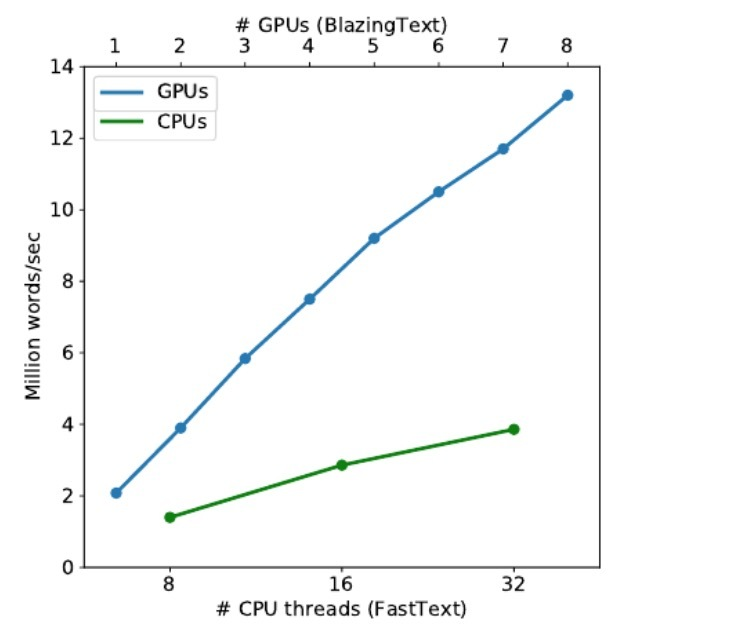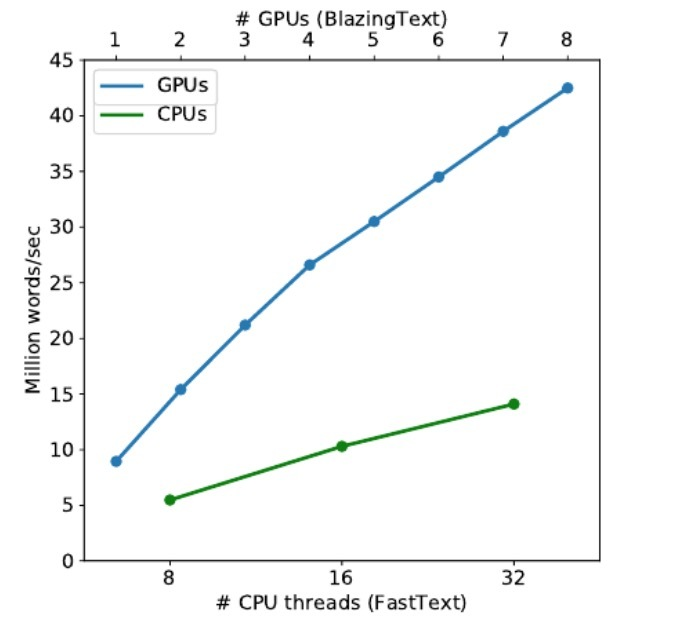## 5.2 Accuracy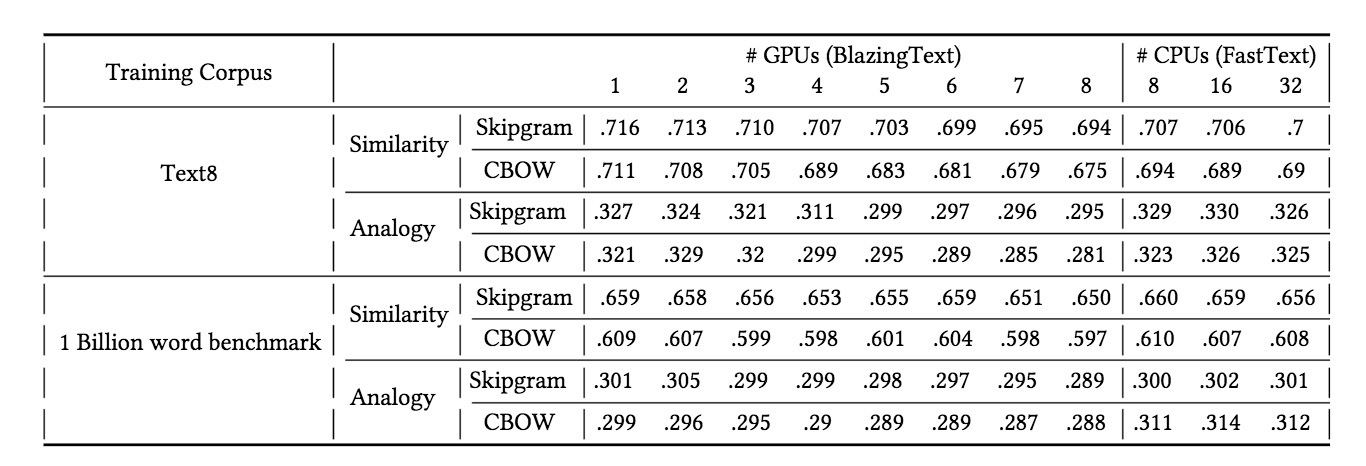# 3. gcForest算法## 3.2 Multi-Grained Scanning

DNN在处理特征关系上很强大，例如，CNN在图片数据上很有效（其中原始像素间的空间关系是很重要的）；RNN对于序列型数据很有效（其中序列关系很重要）。受它们的启发，gcForest使用滑动窗口（sliding windows）来扫描原始特征。假设有400个原始特征，我们使用100个特征的window size。对于序列数据，可以通过对每隔一个特征进行窗口滑动来生成一个100维的特征向量；总共可以生成301个特征向量。如果原始特征具有空间关系，比如：在400个图片像素上的20x20的panel，接着一个10x10的window可以产生121个特征向量（比如：121 10x10 panels）。所有的特征向量从正负训练样本上被抽取（忽略正负），接着被用于生成像3.1所述的类向量：从相同size的window中抽取的样本会被用于训练一个completely-random tree forest和一个 random forest，接着生成的类向量被串联作为转换后的特征。如图4所示，假设存在3个类和使用100维的window，对应于一个400维的原始特征向量，会产生一个1806维的转换特征向量。# 4.实验

## 4.6 Multi-Grained Scanning的影响# 参考

https://arxiv.org/pdf/1702.08835.pdf